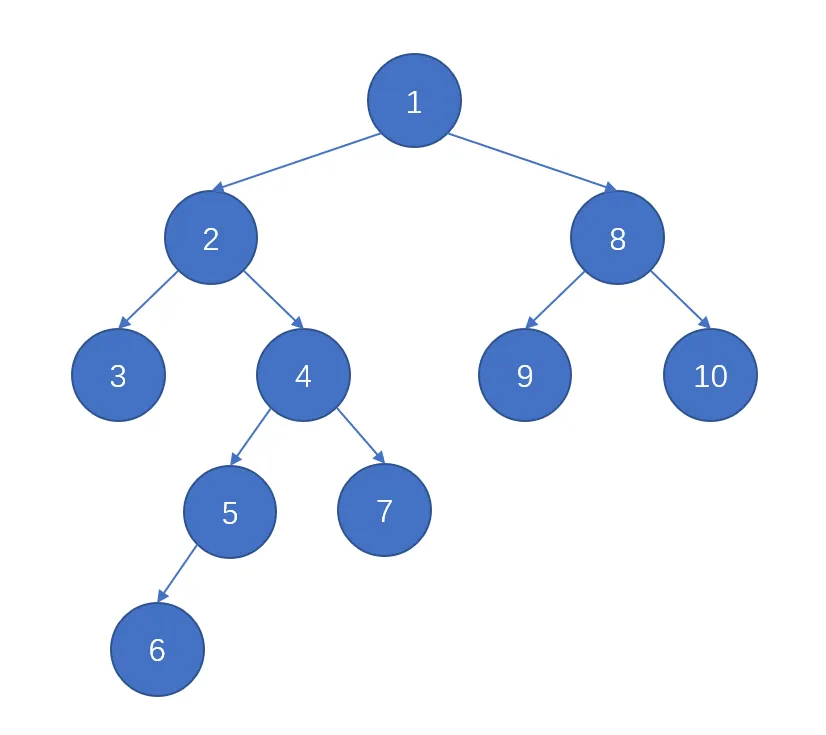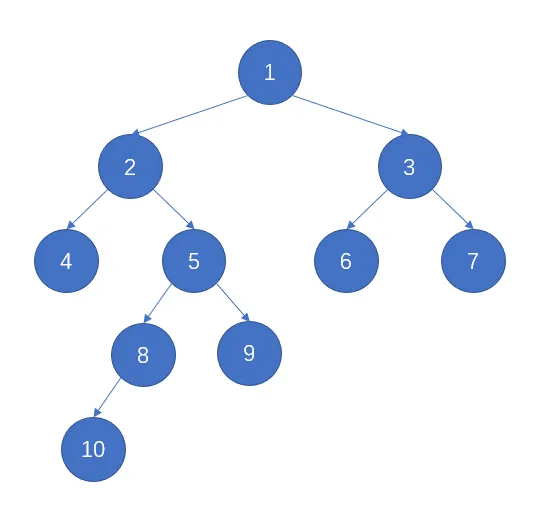# 深度优先搜索 (DFS)

## 搜索方式• 向深搜索 $1\rightarrow2\rightarrow3$，无路可走。
• 回溯一步 $2\leftarrow3$，有新的路线。
• 向深搜索 $2\rightarrow4\rightarrow5\rightarrow6$，无路可走。
• 回溯一步 $5\leftarrow6$，没有新路线，再回溯一步 $4\leftarrow5$，有新的路线。
• 向深搜索 $4\rightarrow7$，无路可走。
• 回溯一步 $4\leftarrow7$，没有新路线，再回溯一步 $2\leftarrow4$，没有新路线，再回溯一步 $1\leftarrow2$，有新的路线。
• $\cdots\cdots$
• 所有节点遍历完成

## 时空复杂度

DFS 俗称暴力搜索，因为它遍历了所有可能情况，因此它的时间复杂度较高，能处理的数据规模较小。

## 代码模板

type dfs(type x, ... ) // 可以存在多个变量
{
if( ... ) // 达成目标，找到答案
{
... // 输出答案或判断最优解等等
return;
}
if( ... ) // 达到搜索边界（即到边界了还没搜到，有时没有此步骤）
{
return;
}
for( ... ) // 遍历所有子节点
{
if( ... ) // 可以转移状态，一般用标志变量判断
{
... // 修改标志变量，表明此节点不可转移
dfs( ... ) // 搜索子节点，经常为x+1
... // 还原标志变量，表面此节点可转移
}
}
}

# 广度优先搜索 (BFS)

## 搜索方式• 遍历深度为 $1$ 的节点 $1$
• 遍历深度为 $2$ 的节点 $2,3$
• 遍历深度为 $3$ 的节点 $4,5,6,7$
• 遍历深度为 $4$ 的节点 $8,9$
• 遍历深度为 $5$ 的节点 $10$
• 所有节点遍历完成

## 时空复杂度

BFS 也可以将所有情况遍历到，那么时间复杂度和 DFS 差不多。但 BFS 我们往往找到答案就会终止，并且可能更快找到答案，因此有时时间复杂度比 DFS 更好。

BFS 在每层遍历时需要保存该层所有节点，因此空间复杂度较高。

## 代码实现模板

bool vis[MAXN]; // 标记是否搜索过，有时也可直接用depth来判断
int depth[MAXN]; // 储存搜索深度，有时可能为二维数组或map
queue<type> que; // STL队列，不过数组模拟队列效率更高

type bfs(type start)
{
que.push(start); // 起点入队
depth[start] = 0; // 起点深度0
vis[start] = true; // 标记起点
while (!que.empty())
{
type now = que.front(); // 当前节点设置为队首
que.pop(); // 弹出队首
if ( ... ) // 如果达到目标条件
{
ans = depth[now]; // 储存答案
return; // 搜索结束
}
for( ... ) // 遍历now节点的所有子节点，可用数组表示方向
{
type next = ... // 计算出子节点
if (!vis[next] && ... ) // 如果子节点未搜索过，且范围符合题目条件
{
vis[next] = true; // 标记子节点
depth[next] = depth[now] + 1; // 子节点深度+1
que.push(next); // 子节点入队
// 有时题目还需输出具体路径，可用一个数组储存每个节点的上一个节点，然后在此处对数组赋值。输出时，从结尾递归反向输出即可获得具体的路径。
}
}
}
}

## 典型题型

POJ1077 - Eight: http://poj.org/problem?id=1077

POJ3984 - 迷宫问题: http://poj.org/problem?id=3984